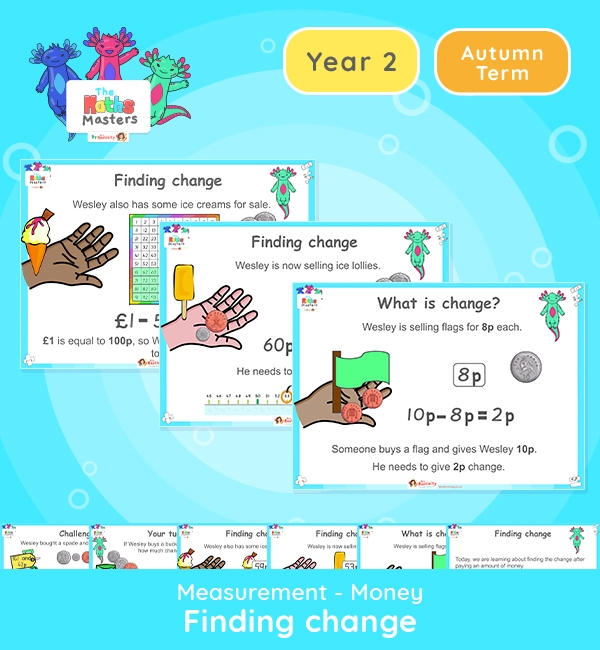# Year 2 | Find Change (Money) Lesson Presentation## Year 2 money resources

Aligned with the maths mastery approach, this Year 2 | Find Change (Money) Lesson Presentation is fully editable, and is designed for the Year 2 maths curriculum covering the following maths objectives for the autumn term:

Topic: Measurement – Money

This lesson focuses on working out finding change from a given amount of money.

Small Steps: Find change

NC Links: Recognise and use symbols for pounds (£) and pence (p); combine amounts to make a particular value *Solve simple problems in a practical context involving addition and subtraction of money of the same unit, including giving change.

TAF Statements: Working Towards – Know the value of different coins

Working At: Use different coins to make the same amount.

Greater Depth – Use reasoning about numbers and relationships to solve more complex problems and explain their thinking.

2AS-2 Recognise the subtraction structure of ‘difference’ and answer questions of the form “How many more….?”

2AS-3 Add and subtract within 100 by applying related one-digit addition and subtraction facts: add and subtract only ones or only tens to/from a two-digit number

2AS-4 Add and subtract within 100 by applying related one-digit addition and subtraction facts: add and subtract any 2 two-digit numbers

Explore our other  year 2 money resources.

## Recently Viewed